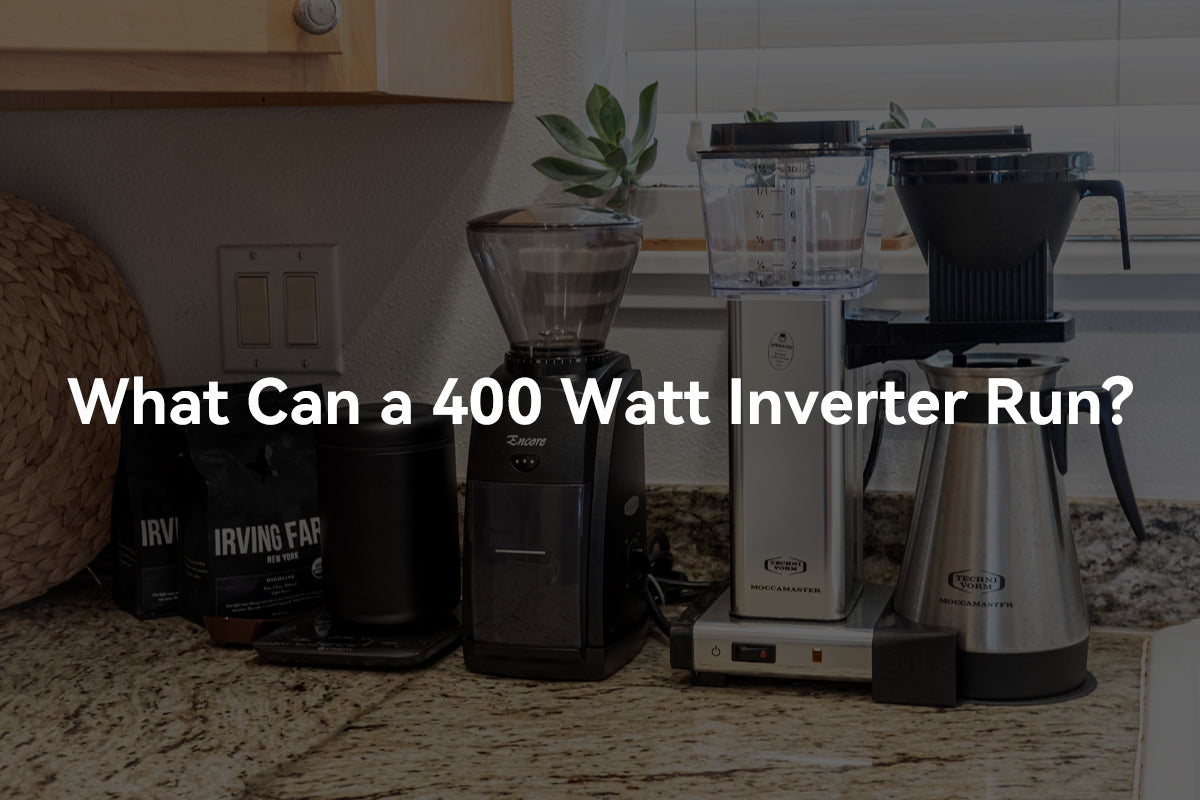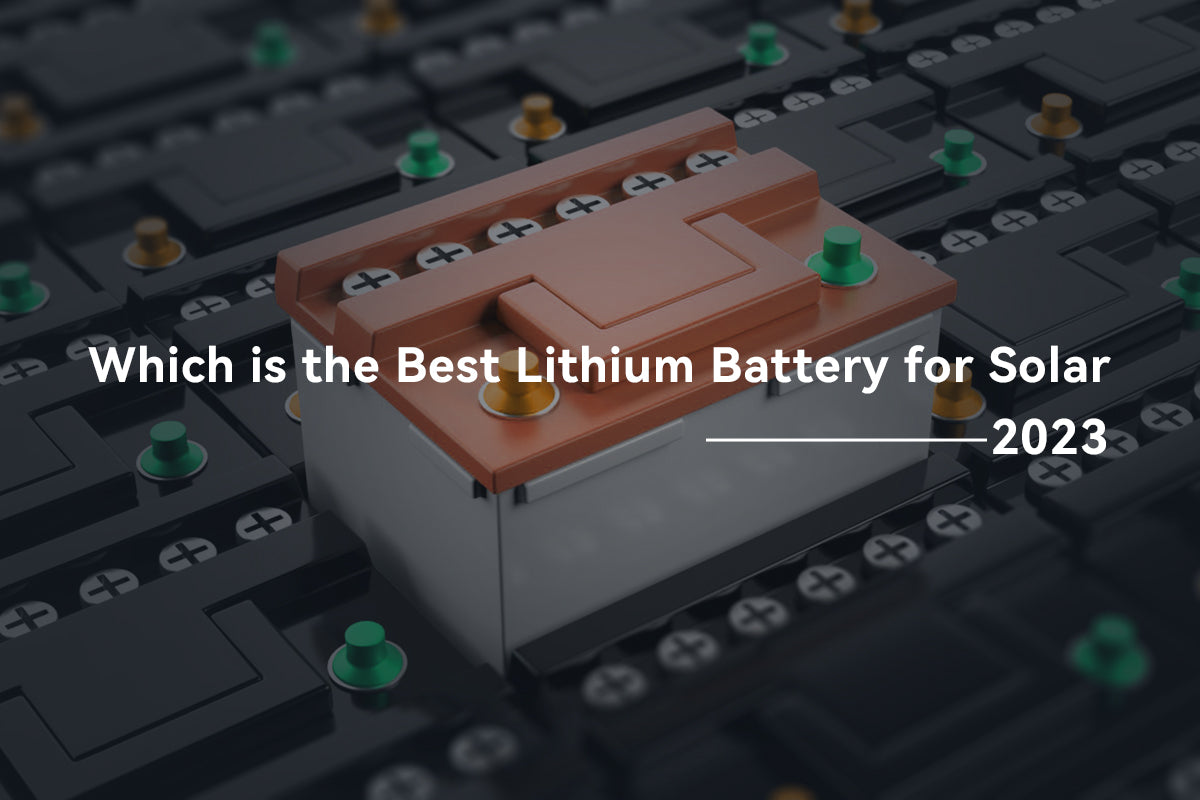# What Can a 400 Watt Inverter Run? - PowMrWhat can a 400 Watt inverter run? First and foremost, you need to have an understanding of the power consumption equipment and the power of the inverter. So in the beginning we will introduce you to the concept of peak power and power rating, and then explain what can be powered by a 400 watt inverter.

## Inverter basic - peak power vs rated power

When selecting an inverter, it's important to consider both the peak power and rated power of the system, and choose an inverter that has a suitable power rating and is compatible with the system's power requirements. This can help ensure optimal performanceefficiency, and safety of the system.

### What is peak power

Peak power refers to the maximum amount of power that a device, machine, or system can generate or consume in a given period of time. It is usually measured in watts (W) or kilowatts (kW) and is typically used to describe the maximum power output of an electrical device, such as a generator, motor, or amplifier.

If you want to power an inductive load with the 400 watt inverter, the peak power should be especially taken into account.

For example, if a generator is rated at 400 watts, it can produce a output slightly higher than 400W for a limited period of time, usually a few seconds or minutes, before its performance begins to degrade.

### What is continuous/rated power

Continuous/rated power refers to the amount of power that an electronic device or system can deliver continuously over an extended period without overheating or failing. It is the maximum power that a device or system can handle without sustaining damage, and is typically expressed in watts (W). This value is typically specified by the manufacturer and is used to determine the capacity and capabilities of the device or system.

For example, The "400 watt" rating means that the inverter can deliver a maximum power output of 400 watts. This rating is important because it determines the maximum load that can be connected to the inverter without causing it to overload or fail.

It's important to note that the actual power output of the inverter may vary depending on the input voltage, temperature, and other factors.

Additionally, the inverter should not be used to power devices that require more power than its rated output, as this can cause damage to the inverter or the connected devices.

## What will a 400 watt inverter run?

A 400watt power inverter can run any number of devices as long as the total power of the loads does not exceed 400 watts and the peak power of the load remains within the power inverter's surge power capacity.

Generally speaking, a 400 Watt power inverter can run many household devices, such as heater, small televisions, laptops, cell phones, digital cameras, portable stereos, small power coffee makers, small power refrigerators (car refrigerators), and other electrical devices that are not very powerful.

### Can a 400 Watt inverter run a TV?

In general, most modern flat-screen TVs consume between 50 and 150 watts of power.

If your TV consumes 50 watts or less, then a 400-watt inverter should be able to run it without any issues. However, if your TV consumes more than 50 watts, you may experience problems with the inverter.

It's also worth noting that while a 400-watt inverter may be able to handle the power requirements of your TV, it may not be enough to power any additional devices at the same time, such as a DVD player or game console.

### Will a 400 watt inverter run a refrigerator

The answer to this question depends on the power requirements of the refrigerator you want to run.

Typically, refrigerators require a high surge of power to start up, which is known as the starting or peak power. This surge can be two to three times the normal running power of the refrigerator.

A 400-watt inverter may be able to power a small refrigerator that requires less than 400 watts of continuous power, but it may not be able to handle the surge power required to start the refrigerator.

Therefore, it is important to check the power requirements of your refrigerator and choose an inverter that can handle both the continuous and peak power requirements of the appliance. It is also recommended to add a safety margin to ensure the inverter can handle any unexpected power spikes or surges.

## How many amps is 400Watts?

To determine the amperage for a given wattage, you need to know the voltage at which the power is being supplied. Amperage is calculated by dividing the power (in watts) by the voltage (in volts).

If we assume a standard voltage of 120 volts in this example, we can calculate the amperage for 400 watts:

• Amperage = Power (watts) / Voltage (volts)

• Amperage = 400 watts / 120 volts ≈ 3.33 amps

Therefore, at 120 volts, 400 watts is approximately equal to 3.33 amps.

## How many batteries do 400 watts take?

The number of batteries required to produce 400 watts of power depends on several factors, such as the capacity and voltage of the batteries, the efficiency of the system, and the duration of the power needed.

However, we can make an estimation based on some assumptions. Let's assume that we are using 12-volt lead-acid batteries with a capacity of 100Ah (amp-hours).

To determine how many batteries are needed, we first need to convert the power (in watts) to energy (in watt-hours) by multiplying it by the time (in hours) the batteries need to provide power.

For example, if we need to power a device for 2 hours, we need 800 watt-hours (400 watts x 2 hours) of energy.

Next, we need to divide the total energy required by the voltage of the batteries. In our case, 800 watt-hours / 12 volts = 66.67 Ah (amp-hours).

Since each battery has a capacity of 100Ah, we would need at least one battery to provide the required energy.

However, it's important to note that this calculation is a simplification and doesn't take into account factors such as battery efficiencydischarge rates, and the impact of temperature on battery performance.

Therefore, it's always best to consult a professional or use a battery sizing calculator to ensure that you have the appropriate number and type of batteries for your specific application.

## How long can a 100Ah lithium battery-run an appliance that requires 400W?

To calculate how long a 100Ah lithium battery can run an appliance that requires 400W, we need to use the following formula:

• Battery Life = Battery Capacity / Device Power

Where:

• Battery Life = the number of hours the battery can run the device

• Battery Capacity = the capacity of the battery, in ampere-hours (Ah)

• Device Power = the power consumption of the device, in watts (W)

So, in this case, we have:

• Battery Life = 100Ah / 400W = 0.25 hours

Therefore, a 100Ah lithium battery can run an appliance that requires 400W for approximately 0.25 hours, or 15 minutes, before it needs to be recharged.

Note:
The length of time the batteries will last with a 400 Watt inverter depends on several factors, including the capacity of the batteries, the efficiency of the inverter, and the amount of power being drawn by the devices connected to the inverter.

## Do I need a fuse between the battery and the inverter?

Yes, a fuse is needed between the battery and the inverter to prevent a fire. If your AC/DC inverter short-circuits, the fuse between it and the battery will prevent the battery from potentially exploding and will cut the circuit fast enough to prevent the wires from catching fire or overheating.

### What size fuse do I need for a 400 watt inverter?

Inverter Voltage Continuous Watts Fuse Size (DC Amps)
12V 2500 400
2800 400
3000 400
24V 600 50